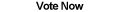Home Articles Components Code Snippets Links

C# Code Snippets# C# Combination Function

Visual C# Kicks

Calculates the number of combinations from choosing r elements from a set of n total elements. The function takes advantage of the fact that the number of combinations is symmetric (based on r) to avoid calculating large numbers when possible.

Platform: .NET Framework 2.0

```public long Factorial(long x, long lowerBound)
{
long fact = 1;
while (x >= 1 && x > lowerBound)
{
fact *= x;
x--;
}
return fact;
}

public long Choose(long n, long r)
{
return (long)((double)Factorial(n, Math.Max(n - r, r)) / (Factorial(Math.Min(n - r, r))));
}
```

Back to C# Code Snippet List

Other C# Articles